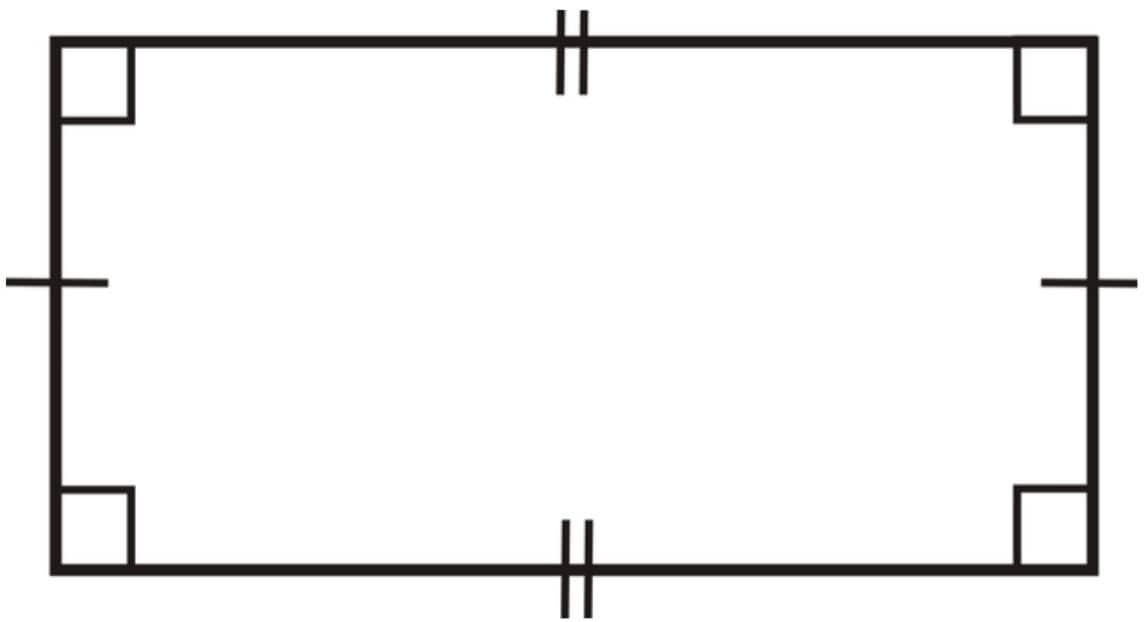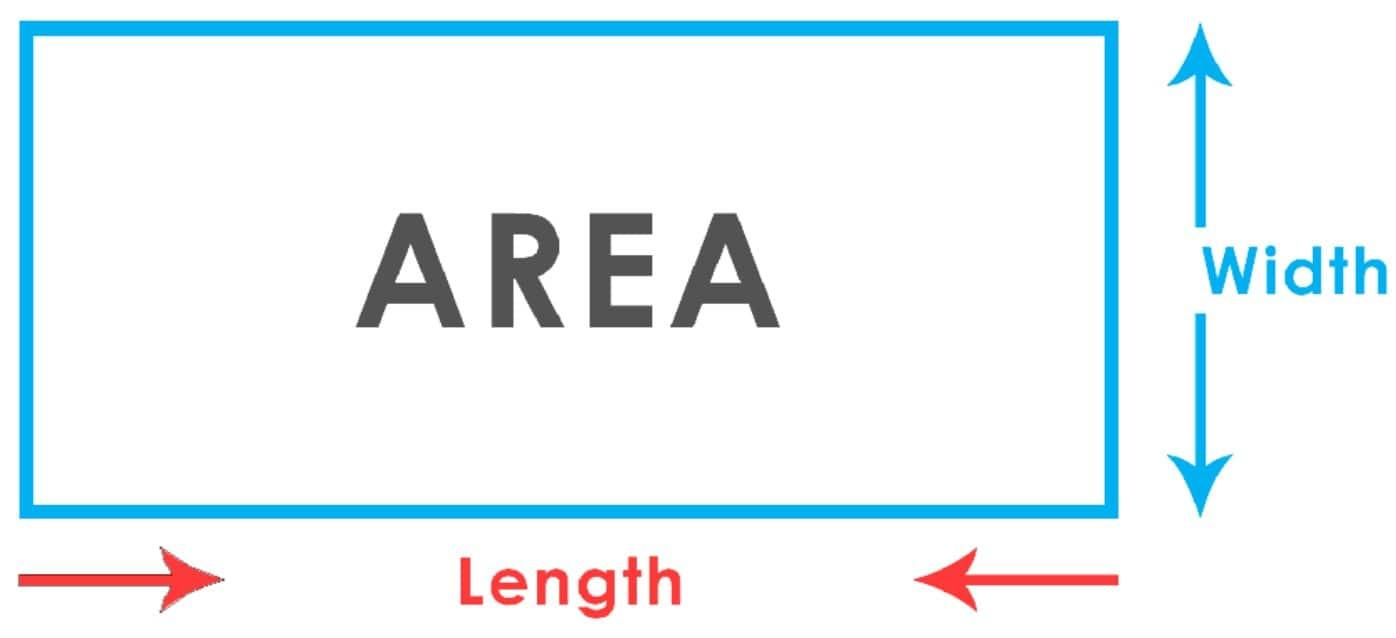Area of Rectangle

Area of Rectangle - Definition, Formula and Problems

Any closed shape made up of at least three-line segments is known as a polygon. There are many different types of polygons, some of them being triangles, quadrilaterals, pentagons hexagons etc. The polygons are classified by the number of edges they have. Edges are also called sides, and they are nothing but the line segments which make up the polygons. The points where the line segments intersect are called vertices. A polygon has an equal number of edges and vertices.

Polygons are classified based on the number of edges or vertices they have. A polygon with four sides is called a quadrilateral or tetragon. Quadrilaterals can be further classified into concave quadrilaterals, convex quadrilaterals, and right-angled quadrilaterals based on the angles they contain. If all four sides of the quadrilateral are 90°, they are right-angled quadrilaterals. They are also called equiangular quadrilaterals since all their angles are equal. The two types of equiangular quadrilaterals are squares and rectangles. In a square all the four sides of the quadrilateral are equal. In a rectangle, only the opposite sides of the quadrilateral are equal.Since the opposite sides of the square are equal, the square can also be considered as a rectangle. The rectangle is also a parallelogram, which means the opposite sides of the rectangle are perfectly parallel to each other. We can also say that a rectangle with all four sides equal to each other is a square.

Area of a Rectangle:

The area of any polygon is the amount of space it occupies or encloses. It is the number of square units inside the polygon. The area is a two-dimensional property, which means it contains both length and width. The area is usually measured in units like square meters, square feet, or square inches. Area of larger shapes, like fields or cities is measured in square kilometres, hectares, or acres.

To find the area of a rectangle, we simply need to multiply its length by its width.The area is simply the width of a rectangle times height.

The formula to calculate the area of a rectangle is given as
Area = w × h

For example, if a rectangle is 8 m wide and 3 m high,
the area of the rectangle is Area = w × h
Area = 8 × 3
Area = 24 m2

In a square, since all the four sides are equal, Area = w × h becomes

Area = h × h, Area = h2.

Hence for a square, the area is the square of its sides.

Problems relating to the area of a Rectangle:

• 1) Find the area of a rectangle whose height is 20 cm and width is 4 cm.

• Solution: Given, Height = 20 cm and Width = 4 cm
Area of a rectangle = w × h
Area of a rectangle = 20 × 4
Therefore, the area of the rectangle = 80 cm2.
• 2) A rectangular board has a width of 120 mm and a height of 89 mm. Find the area of this board.

• Solution: Given, Height = 89 mm = 8.9 cm, and Width = 120 mm = 12 cm
Area of a rectangle = w × h
Area of a rectangle = 12 × 8.9
Therefore, the area of the rectangle = 106.8 cm2.

• 3) The height of a rectangular screen is found to be 20 cm. Its area is found to be 240 cm2. Find the width of the given screen.

• Solution: Given, Height = 20 cm and Area = 240 cm2.
Area of a rectangle = w × h
Therefore, Width = Area / length
Width = 240/20
Width = 120 cm

• 4) The height and width of a rectangular wall are 80 m and 60 m respectively. If a painter charges ₹ 3 per m2 for painting the wall, how much would it cost to have the whole wall painted?

• Solution: Given, Height = 80 m and Width = 60 m
Area of a rectangle = w × h
Area of a rectangle = 80 × 60
Area of a rectangle = 4800 m2
At the cost of ₹ 3 per m2, the cost for painting 4800 m2 is
4800 × 3 =₹ 14,400

• 5) A floor whose length and width are 60 m and 40 m respectively need to be covered by rectangular tiles. The dimension of the selected floor tiles is 1 m × 2 m. Find the total number of tiles needed to fully cover the room.

• Solution: Given, Length = 60 m and Width = 40 m
Area of a rectangle = w × h
Area of a rectangle = 60 × 40
Area of the rectangle = 2400 m2
The length of one tile = 1m
The width of one tile = 2 m
Area = w × h
Area of one tile = 1 × 2
Area of one tile = 2 m2
Number of tiles required for covering the floor can be counted by
Area of floor / Area of a tile
2400/2 = 1200 tiles.

• 6) A square piece of cardboard with the area equal to 36 cm2 is cut out from a rectangular piece of cardboard. Find

• (a) The length of the square
(b) If the cardboard can be cut into three such squares, find the length, breadth, and area of this rectangular piece of cardboard.

Solution: (a) Given, The area of the square is 36 cm2
We know that in a square, Area = h2.
Therefore, the length of the square = √Area
Length = √36
Length = 6 cm
Solution: (b) Given, three squares of length 6 cm can be cut from the cardboard
Therefore, height of the piece of cardboard = 6 cm
Width of the piece of cardboard = 6 + 6 + 6 = 18 cm
Since length = 6 cm and width = 18 cm,
Area = w × h
Area = 6 × 18
Area = 108 cm2.